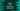# Python program to find larger string among two strings## Python program to find larger string among two strings:

In this tutorial, we will learn how to find the larger string among two user input strings in python. One string is called larger than another string if its length is greater than the length of the other string. So, we need to find out the length of a string if we want to compare it with another.

With this tutorial, you will learn how to find the length of a string and how to compare two values in python. The user will enter two strings, our program will read them both and without using any inbuilt function, it will calculate the length of each string. It will then print out the larger string to the user. I will also show you how to find the length of a string easily by using an inbuilt function at the end of this tutorial.

Let’s take a look at the program first :

### Python program to find larger string:

``````#1
first_string = input("Enter the first string : ")
second_string = input("Enter the second string : ")

length1 = 0
length2 = 0

#2
for i in first_string:
length1 = length1 + 1

#3
for i in second_string:
length2 = length2 + 1

#4
if length1 > length2 :
print("First string is larger than the second")
else :
print("Second string is larger than the first")``````

You can find the source code here.

### Explanation:

The commented number in the above program denotes the step number below :

1. Ask the user to enter the first string. Read it and save it in the firststring_ variable. Similarly, read the second string and save it in the secondstring_ variable. The program will compare these two strings. For reading the user input string, we are using the input() method. We are also declaring two variables ‘length1’ and ‘length2’ to hold the length of the first and the second string.
2. To calculate the length of a string, we are using one for loop here. It scans each character of the string. For each character, increment the value of length1 by 1. length1 is holding the size of the first string. After the for loop will complete, length1 will hold the length of the first string.
3. Similarly, calculate the length of the second string and store the length in variable length2. We are using one for loop similar to the above.
4. After the lengths are calculated, check which one is greater. Also, print out the message accordingly. For this step, we are using one if-else condition. If the length of the first string is greater than the second string, print statement inside the if statement will execute. Else, the print statement inside the else statement will execute.

## Another way to find the length of a string :

Instead of using a for loop, we can also use len() method to find out the length of a string. The syntax of the method is as below :

``len(str)``

As you can see, we need to pass one string as its parameter. It will calculate the length of the parameter string and returns the value.

The return length value is of integer type. We don’t need to import any module to use this method.

Try to change the above program to use len() instead of running a for-loop for each string.

### Conclusion :

In this tutorial, we have learned how to find the larger string among two in python. You can extend this program to find out the larger string among ’n’ different strings. Instead of using a for-loop, use len() method to find out the length. len() will reduce the size of the program.